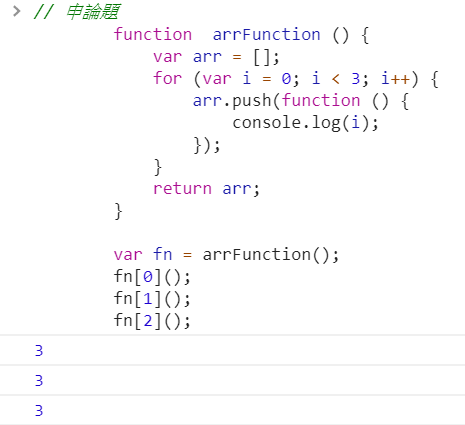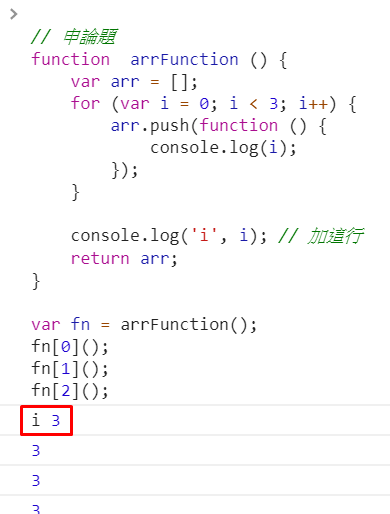0

# 首先我們先來看看下面的例子

``````// 申論題
function  arrFunction () {
var arr = [];
for (var i = 0; i < 3; i++) {
arr.push(function () {
console.log(i);
});
}
return arr;
}

var fn = arrFunction();
fn();
fn();
fn();
````````````// 申論題
function  arrFunction () {
var arr = [];
for (var i = 0; i < 3; i++) {
arr.push(function () {
console.log(i);
});
}

console.log('i', i); // 加這行
return arr;
}

var fn = arrFunction();
fn();
fn();
fn();
````````````// 申論題
function  arrFunction () {
var arr = [];
for (var i = 0; i < 3; i++) {
(function (j) { // 改成 j
arr.push(function () {
console.log(j); // 改成印出 j
});
})(i); // 每次累加的 i 都傳入立即函式中
}
return arr;
}

var fn = arrFunction();
fn();
fn();
fn();
``````

``````// 申論題
function  arrFunction () {
var arr = [];
for (let i = 0; i < 3; i++) {
arr.push(function () {
console.log(i);
});
}
return arr;
}

var fn = arrFunction();
fn();
fn();
fn();
``````

``````function storeMoney() {
var money = 1000;
return function (price) {
money = money + price;
return money;
}
}
``````

``````// 函式工廠
function storeMoney (initValue) {
var money = initValue || 1000;
return function (price) {
money = money + price;
return money;
}
}

var MingMoney = storeMoney(100);
console.log(MingMoney(500));
``````

``````// 函式工廠
function storeMoney (initValue) {
var money = initValue || 1000;
// 私有方法
return {
increase: function (price) {
money += price;
},
decrease: function (price) {
money -= price;
},
value: function (price) {
return money;
}
}
}
``````

``````var MingMoney = storeMoney(100);

MingMoney.increase(100);
MingMoney.increase(100);
MingMoney.increase(300);
MingMoney.increase(290);

MingMoney.decrease(310);
console.log(MingMoney.value());
``````

0